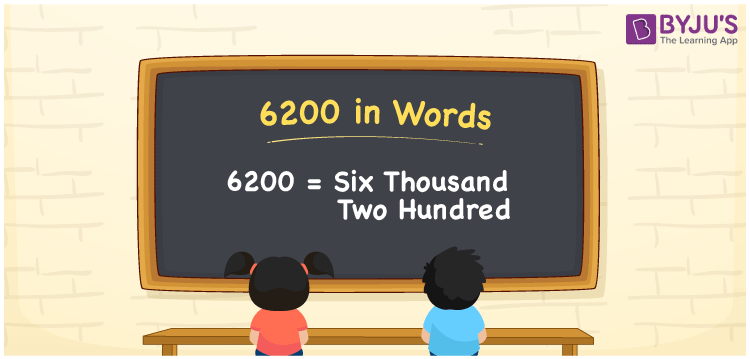# 6200 in Words

We can write 6200 in words as Six Thousand Two Hundred. Suppose you have Rs. 6200 in your bank account, you can say that “I have Six Thousand Two Hundred rupees in my bank account”. It is possible to convert 6200 into words with the help of a place value chart. Also, we know that 6200 is a cardinal number since it represents a specific quantity.

 6200 in words Six Thousand Two Hundred Six Thousand Two Hundred in Numbers 6200

## 6200 in English Words## How to Write 6200 in Words?

We can convert 6200 to words using a place value chart. This can be done as follows. The number 6200 has 4 digits, so let’s make a chart that shows the place value up to 4 digits.

 Thousands Hundreds Tens Ones 6 2 0 0

Thus, we can write the expanded form as:

6 × Thousand + 2 × Hundred + 0 × Ten + 0 × One

= 6 × 1000 + 2 × 100 + 0 × 10 + 0 × 1

= 6200

= Six Thousand Two Hundred

6200 is the natural number that is succeeded by 6199 and preceded by 6201.

6200 in words – Six Thousand Two Hundred

Is 6200 an odd number? – No.

Is 6200 an even number? – Yes

Is 6200 a perfect square number? – No

Is 6200 a perfect cube number? – No

Is 6200 a prime number? – No

Is 6200 a composite number? – Yes

## Frequently Asked Questions on 6200 in Words

Q1

### How to write 6200 in words?

6200 in English is written as “Six Thousand Two Hundred”.
Q2

### Is 6200 a prime number?

No, the number 6200 is not a prime number. Six Thousand Two Hundred is a composite number. Because 6200 has more divisors than 1 and itself.
Q3

### Write Six Thousand Two Hundred in numbers.

Six Thousand Two Hundred in numbers is 6200.